• import numpy as np a = np.array(1,10) a = a.reshape(3,3) ...#对多维数组切片，切出前两个行的前两个列 print(a[:2,:2]) #切出1，3行的1，3列 print(a[::2,::2]) #切片赋值 a[::2,::2] = 99 pr...
import numpy as np

a = np.array(1,10)
#将ndarray数组赋值变维成3行3列的数组
a = a.reshape(3,3)
print(a,a.shape)	#[[1 2 3][4 5 6][7 8 9]] (3,3)
#对多维数组切片，切出前两个行的前两个列(切片是对其维度进行切片)
print(a[:2,:2])
#切出1，3行的1，3列
print(a[::2,::2])

#切片赋值
a[::2,::2] = 99
print(a)	#[[99 2 99][4 5 6][99 8 99]]
展开全文numpy
• Python 3.7.4 (tags/v3.7.4:e09359112e, Jul 8 2019, 20:34:20) [MSC v.1916 64 bit (AMD64)] on win32 ...>>> import numpy as np >>> a = np.zeros((3,5)) >>> a array
Python 3.7.4 (tags/v3.7.4:e09359112e, Jul  8 2019, 20:34:20) [MSC v.1916 64 bit (AMD64)] on win32
>>> import numpy as np
>>> a = np.zeros((3,5))
>>> a
array([[0., 0., 0., 0., 0.],
[0., 0., 0., 0., 0.],
[0., 0., 0., 0., 0.]])
>>> b = np.ones((3,5))
>>> b
array([[1., 1., 1., 1., 1.],
[1., 1., 1., 1., 1.],
[1., 1., 1., 1., 1.]])
>>> a[:2] = b[:2,:]
>>> a
array([[1., 1., 1., 1., 1.],
[1., 1., 1., 1., 1.],
[0., 0., 0., 0., 0.]])
>>>
>>> a = np.zeros((3,5))
>>> a[:2,:3] = b[:2,:3]
>>> a
array([[1., 1., 1., 0., 0.],
[1., 1., 1., 0., 0.],
[0., 0., 0., 0., 0.]])
>>>
>>> a = np.zeros((3,5))
>>> a[::2] = b[::2]
>>> a
array([[1., 1., 1., 1., 1.],
[0., 0., 0., 0., 0.],
[1., 1., 1., 1., 1.]])
>>>
>>>
>>>

展开全文• 1. 多维数组切片 定义Numpy的一个多维数组 import numpy as np ArrOne = np.arange(20).reshape(4,5) # np.arange(20),生成0-19，20长一维向量，通过reshape变成4*5的二维数组 print("ArrOne ->> ", '\n', ...

1. 多维数组的切片

定义Numpy的一个多维数组

import numpy as np
ArrOne = np.arange(20).reshape(4,5)
# np.arange(20),生成0-19，20长一维向量，通过reshape变成4*5的二维数组
print("ArrOne ->> ", '\n', ArrOne)
print("ArrOne的维度 ->> " , ArrOne.shape)

输出如下：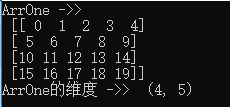假如我们要取出红框中的元素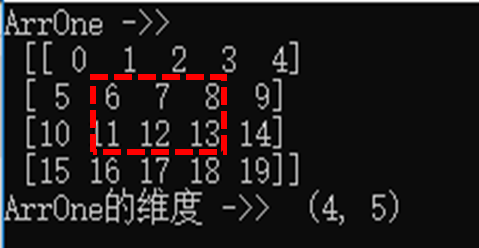红框中的数据是矩阵ArrOne第2，3行与第2,3，4列包围的数据.，使用两种方法取出数据：

RedBoxArr_1 = ArrOne[1:3, 1: 4]
RedBoxArr_2 = ArrOne[1: 3, :][:, 1: 4] #前一个冒号可以省略，后一个不能
print("RedBoxArr_1 ->> ", '\n', RedBoxArr_1)
print('ArrOne[1: 3]','\n', ArrOne[1: 3])
print("RedBoxArr_2 ->> ", '\n', RedBoxArr_2)
## RedBoxArr_2等价于：
## a = ArrOne[1: 3]
## RedboxArr_2 = a[1: 4]

输出如下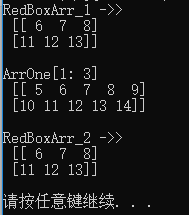可以看到用这两种方法都可以实现多维数组的切片。

2. 注意事项

要注意：从多维数组中取出某一列，造成的维度变化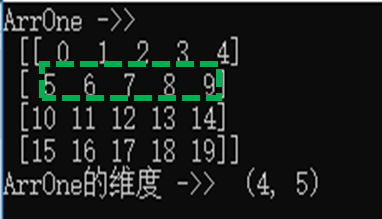如要取出ArrOne第2行的元素，代码阐述：

GreeBoxArr_1 = ArrOne
GreeBoxArr_2 = ArrOne[1:2]
# 我们看看有什么区别
print('GreeBoxArr_1', '\n', GreeBoxArr_1, '\n')
print('GreeBoxArr_2','\n',  GreeBoxArr_2, '\n')
print('GreeBoxArr_1\'s shape', '\n', GreeBoxArr_1.shape, '\n')
print('GreeBoxArr_2','\n',  GreeBoxArr_2.shape, '\n')

输出结果如下：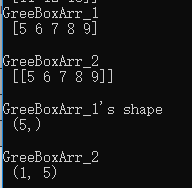我们看到这两种方式都取出了ArrOne第2行的元素。但是用第一种取出的只有一个维度。而用第二种方法取出的有两个维度。在进行矩阵运算时一定要注意这两者取值的差异。
结论：Arr[1:2],Arr[:, 1:2]等方式切片能够保持矩阵维度在2维（取决Arr本身的维度），而用Arr, Arr[:, 1]方式切片不能够维持原本的矩阵维度。
计算demo：

ArrTwo = np.arange(5).reshape(5, 1)
ArrThree = np.arange(5).reshape(1, 5)
ResultOfGreeBox1_1 = np.matmul(ArrTwo, GreeBoxArr_1)
ResultOfGreeBox1_2 = np.matmul(ArrThree, GreeBoxArr_1)
ResultOfGreeBox2_1 = np.matmul(ArrTwo, GreeBoxArr_2)
ResultOfGreeBox2_2 = np.matmul(ArrThree, GreeBoxArr_2)
print('ResultOfGreeBox1_1', '\n', ResultOfGreeBox1_1, '\n')
print('ResultOfGreeBox1_2','\n',  ResultOfGreeBox1_2, '\n')
print('ResultOfGreeBox2_1', '\n', ResultOfGreeBox2_1, '\n')
print('ResultOfGreeBox2_2','\n',  ResultOfGreeBox2_2, '\n')

可以看到ResultOfGreeBox1_1出现了维度异常错误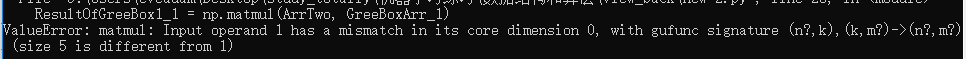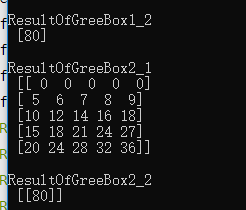展开全文numpy 多维数组切片
• import numpy as np b=np.arange(24) a=b.reshape(2,3,4) print a print a[0,:,0] 2、取所有二维数组下的每个二维数组的第0个元素（一维数组） b=np.arange(24) a=b.reshape(2,3,4) print a print '---------------...
• b数组如上图所示，现在取某一列 从运行结果可以看出，得到的数组变为一行了，而不是一列。特别注意在运算过程中，还要进行变换，可以用 e.reshape(6,1) 就可以了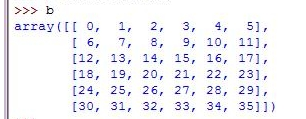b数组如上图所示，现在取某一列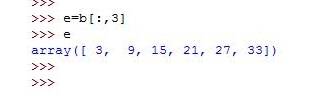从运行结果可以看出，得到的数组变为一行了，而不是一列。特别注意在运算过程中，还要进行变换，可以用
e.reshape(6,1)
就可以了

展开全文python
• 概述numpy多维数组的索引与切片跟python中的列表类似，但最大的区别是，数组切片是原始数组的视图，也就是说对视图上的修改直接会影响到原始数组，因为numpy的主要是处理大数据，如果每次切片都进行一次复制，那对...多维数组的索引与切片
• 参考链接: Structural indexing tools 参考链接: np.clip() 函数 和 numpy切片省略号的用法 参考链接: NumPy 切片和索引
• 多维数组切片和索引 import numpy as np b = np.arange(24) print(b) [ 0 1 2 3 4 5 6 7 8 9 10 11 12 13 14 15 16 17 18 19 20 21 22 23] reshape函数切分的思想，先分两半，对应2，再在每个分3份对应3…… ...numpy python
• numpy多维数组的切片操作

千次阅读 2017-12-11 21:00:23
import numpy as np item = np.array([[1,4,5],[5,3,6],[8,15,33]]) print(item[0,:]) print(item[1,:]) print(item[2,:]) print(item[:,0]) print(item[:,1]) print(item[:,2]) 输出： [1 4 5] [5 3 6] ...
• numpy在处理数据的时候，经常会有切片操作，如提取指定几行的数据或者几列的数据，本文记录一些典型的切片方法以备日后查看。 一维数组切片数组[start????step] 从start到end，以step为步长的元素 且：start &...
• 切片2.1 slice 函数或start:stop:step2.2 省略号‘...’选择数组的维度2.2 多维数组切片2.3 整数数组索引2.4 布尔索引2.5 花式索引 1. 索引 获取数组中特定位置元素的过程。与 Python 中 list 的操作一样，ndarray ...python numpy 索引
• 支持高级大量的维度数组与矩阵运算，此外也针对数组运算提供大量的数学函数库。Numpy内部解除了Python的PIL(全局解释器锁),运算效率极好,是大量机器学习框架的基础库! 简单理解： 　2维是EXCEL表格里面的多行多列...
• import numpy as np np.random.seed(1234) A = np.random.randint(0, 6,(5,3)) #生成10行3列数值在[0,6）的随机数组 print(A) '''结果 [[3 5 4] [4 0 1] [1 1 2] [3 4 4] [2 2 0]] ''' print(A[0:2 , 0:3]) #...python
• import numpy as np a=np.arange(1,37,1)numpy python
• 多维数组和一维数组属性基本相同 索引与切片，赋值替换 拷贝函数 如果不是拷贝函数，对新创建的数组赋值，旧的数组也会发生改变。定义a=b表示内存中的数据同时指向a与b。 数据类型 arange函数 #表示从0-...numpy python
• ndarray的内存实现原理、切片索引的视图原理python numpy 切片索引
• numpy 提供了一种数组类型，高维数组， 提供了数据分析的运算基础（业务表一般就是二维） import numpy as np 导入numpy库，并查看numpy版本 np.version 一、创建Array 1. 使用np.array()由python list创建 C 数组的...
• 一维数组类似MATLAB，多维数组写法不同。... 对一维数组切片或索引核心：操作类似list（），或者MATLAB的一维数组写法输入import numpy as npa = np.arange(10)print('a is:\n',a)# 索引b = aprint(...
• 1、数组运算 数组运算的注意事项： 当使用不同类型的数组操作时，结果数组的类型对应于更一般或更精确的数组，即向上转换，因此不能对低精度的数组元素赋高精度的值； 基本的算术、逻辑、位以及比较运算，需要创建...python numpy ndarray
• 1.修改形状 序号 形状及描述 1. reshape 不改变数据的条件下修改形状 2. flat 数组上的一维迭代器 3. flatten 返回折叠为一维的数组副本 ... ravel 返回连续的...numpy.reshape(arr, newshap...
• python中numpy的数组切片

千次阅读 2018-06-02 23:41:59
一维数组：在numpy的array中切片的操作与python列表的切片操作是相同的，记住索引规则即 a[start,end,step] 二. 二维数组：索引规则为a[行,列]。 例子如下： 先初始化一个二维array import numpy as np a = np....python 编程 numpy 机器学习 深度学习
• Numpy多维数组

2019-09-11 09:47:20
NumPy之美的一个关键部分是它能够将我们目前所看到的所有内容应用到任意数量的维度。 创建矩阵 我们可以传递以下形式的Python列表，让NumPy创建一个矩阵来表示它们： 我们也可以使用上一篇中提到的相同方法 ones()...Numpy多维数组
• 一般的，我们容易误认为通过传入相应索引的数组来直接获取，但是结果出乎我们预期，这时我们得到的却是相应数组构成的坐标对应于ndarray中的元素构成的一维数组。如下所示。 上面例子中，我们想获取arr对象中的右...
• Numpy中，对ndarray数组切片是原始数组的视图，这意味着数据不会被复制，视图上任何的修改都会直接反映到源数组上。 arr = np.arange(10) #[0,1,2,3,4,5,6,7,8,9] arr_slice = arr[5:8] arr_slice = 12345 #...
• senderle..10从语法上讲,这看起来像是一种不一致,...在您的定义中c,您正在进行基本切片,它返回数据视图.为了区分它,有助于理解索引如何传递给python对象.这里有些例子:>>> class ShowIndex(object):... def...
• 其次,元组可能包含切片对象,省略号对象,普通整数或numpy数组. 仅传递int,slice或Ellipsis对象或None(与numpy.newaxis相同)等对象时,将激活基本切片.这些可以单独传递或者在元组中传递.以下是文档关于如何激活基本......